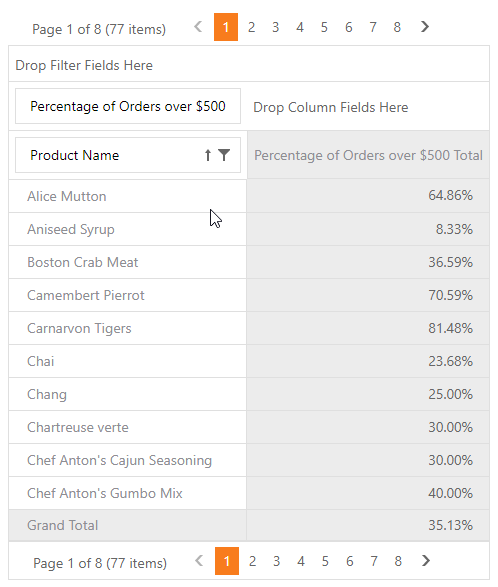19.2
19.1
18.2
18.1
17.2

# ASPxPivotGrid.CustomSummary Event

Enables summary values to be calculated manually.

Namespace: DevExpress.Web.ASPxPivotGrid

Assembly: DevExpress.Web.ASPxPivotGrid.v19.2.dll

## Declaration

``public event PivotGridCustomSummaryEventHandler CustomSummary``
``Public Event CustomSummary As PivotGridCustomSummaryEventHandler``

## Event Data

The CustomSummary event handler receives an argument of the PivotGridCustomSummaryEventArgs type. The following properties provide information specific to this event.

Property Description
ColumnField Gets the column field that corresponds to the current cell.
ColumnFieldValue Gets the value of the column field that corresponds to the current cell.
CustomValue Gets or sets a custom summary value.
DataField Gets the data field against which the summary is calculated.
FieldName Gets the name of the data field against which the summary is calculated.
RowField Gets the row field that corresponds to the current cell.
RowFieldValue Gets the value of the row field that corresponds to the current cell.
SummaryValue Gets an object that contains the values of the predefined summaries which are calculated for the current cell.

## Remarks

The ASPxPivotGrid calculates summaries against data fields. A field's PivotGridFieldBase.SummaryType property specifies the type of summary function. The control automatically calculates all the predefined summary functions (see the PivotSummaryType topic for a list of the available functions) and it allows custom summaries to be calculated manually using the CustomSummary event.

To calculate a custom summary for a specific data field, set its PivotGridFieldBase.SummaryType property to PivotSummaryType.Custom. In this instance, the CustomSummary event fires for each cell that corresponds to this data field. Use the PivotGridCustomSummaryEventArgsBase<T>.DataField property to identify the data field.

To identify the kind of a processed cell, you can use the PivotGridCustomSummaryEventArgsBase<T>.ColumnField and PivotGridCustomSummaryEventArgsBase<T>.RowField properties.

• If ColumnField or RowField is null, it means that the processed cell is a Grand Total.
• If both ColumnField and RowField are last fields in the corresponding area, this means that this is an ordinary cell.
• In other cases, this is a Total cell.

For example, you can handle the CustomSummary event to calculate custom summaries against multiple fields, particular records, etc. Use the PivotGridCustomSummaryEventArgsBase<T>.CreateDrillDownDataSource method to get a list of records that correspond to the processed cell. This list can then be traversed to calculate a custom summary. The custom summary value should be assigned to the PivotGridCustomSummaryEventArgsBase<T>.CustomValue property.

The ASPxPivotGrid calculates all the predefined summaries (AVERAGE, MIN, MAX, SUM, etc.) for each cell. The calculated summaries can be accessed using the PivotGridCustomSummaryEventArgsBase<T>.SummaryValue property and used in custom summary calculations.

##### Note

The CustomSummary is not supported in server and OLAP modes.

## Examples

This example demonstrates different approaches to calculate a custom summary.

The task is to calculate the percentage of units that cost over \$50 and show that value in the total columns.

The following approaches are shown:

### Use CustomSummary Event

A custom summary is calculated for the "Unit Price" field. The field's SummaryType property is set to PivotSummaryType.Custom. The ASPxPivotGrid.CustomSummary event is handled to count the records whose total sum exceeds \$50. The ratio of these records to all the records is assigned to the e.CustomValue parameter.

### Use Unbound Expression

A field is an unbound field with an expression that calculates the ratio.

### Use Data Binding API

In Optimized mode create a field bound to the ExpressionDataBinding object with the following expression:

``````ToDecimal(Sum(iif([Extended_Price]>=500,1,0)))/Count()
``````

The resulting Pivot Grid is shown below:##### Note

The complete sample project How to Implement Custom Summary is available in the DevExpress Examples repository.

``````<dx:ASPxPivotGrid ID="ASPxPivotGrid1" runat="server" DataSourceID="AccessDataSource1" Theme="Office365">
<OptionsData DataProcessingEngine="Optimized" />
<Fields>
<dx:PivotGridField ID="fieldProductName" Area="RowArea" AreaIndex="0"
Caption="Product Name" FieldName="ProductName">
</dx:PivotGridField>
<dx:PivotGridField ID="fieldExtendedPriceNew" Area="DataArea" AreaIndex="0"
Caption="Percentage of Orders over \$500">
<DataBindingSerializable>
<dx:ExpressionDataBinding Expression="ToDecimal(Sum(iif([Extended_Price]>=500,1,0)))/Count()" />
</DataBindingSerializable>
<CellFormat FormatString="p" FormatType="Numeric"></CellFormat>
</dx:PivotGridField>
</Fields>
</dx:ASPxPivotGrid>
``````

``````<dx:ASPxPivotGrid ID="ASPxPivotGrid1" runat="server" DataSourceID="AccessDataSource1"
OnCustomSummary="ASPxPivotGrid1_CustomSummary" Theme="DevEx">
<Fields>
<dx:PivotGridField ID="fieldProductName" Area="RowArea" AreaIndex="0"
Caption="Product Name" FieldName="ProductName">
</dx:PivotGridField>
<dx:PivotGridField ID="fieldExtendedPrice" Area="DataArea" AreaIndex="0"
Caption="Percentage of Orders over \$500" SummaryType="Custom"
CellFormat-FormatString="p" CellFormat-FormatType="Numeric"
FieldName="Extended_Price">
</dx:PivotGridField>
</Fields>
</dx:ASPxPivotGrid>
``````